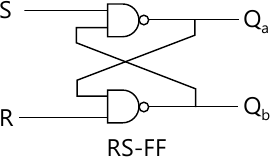MORE IN Digital Electronics and Logic Design
SPPU Computer Engineering (Semester 3)
Digital Electronics and Logic Design
May 2014
Total marks: --
Total time: --
INSTRUCTIONS
(1) Assume appropriate data and state your reasons
(2) Marks are given to the right of every question
(3) Draw neat diagrams wherever necessary

Answer any one question from Q1 and Q2
1 (a) Do the following conversions:
i) (1011.01)2 → ( )10
ii) (4C8.2)16 → ( )10
iii) (o.6234)10 → ( )8.
6 M
1 (b) What is logic family? Give the classification of logic family.
3 M
1 (c) Explain the wired logic output of TTL with neat diagram.
3 M

2 (a) Minimize the following expression using Quine-McClusky:
F(A, B, C, D) = ∑.m (1, 5, 6, 12, 13, 14) + d(2, 4)
6 M
2 (b) Explain with diagram 2 input CMOS NAND gate.
6 M

Answer any one question from Q3 and Q4
3 (a) What do you mean by half adder and full adder ? How will you implement full adder using half adder ? Draw the circuit Diagram.
6 M
3 (b) Explain with neat diagram working of parallel in serial out 4-bit shift register. Draw necessary timing diagram.
6 M

4 (a) Explain in detail Look Ahead Carry generator.
6 M
4 (b) Design a MOD-5 synchronous counter using JK FF and implement it. Also draw timing diagram.
6 M

Answer any one question from Q5 and Q6
5 (a) Draw the ASM chart for the following state machine. A 2-bit up counter is to be designed with output Q, Q0, and enable signal ?X?. If X = 0, then counter changes the state as 00 - 01 - 10 - 11 - 00. If -X- = 1 then counter remains in same state. Design the circuit using JK-FF and suitable MUX.
7 M
5 (b) What is meant by Entity and Architecture in VHDL ? Write the architecture of RS FF as given below in structural modeling. Assume entity NAND 2 A, B input and Y output.6 M

6 (a) Write VHDL code for 2-bit comparator circuit. Use behavioural modeling style.
6 M
6 (b) Draw an ASM chart and state table for a 2-bit Up-Down counter having mode control input M
When M = 1 : UP counting
When M = 0 : Down Counting
The circuit should generate output whenever counter becomes minimum or maximum.
7 M

Answer any one question from Q7 and Q8
7 (a) What do you mean by FPGA ? Explain the internal architecture of FPGA. State the importance of configurable logic block in FPGA.
7 M
7 (b) What is PLA ? Explain the input buffer AND and OR Matrix in PLA.
6 M

8 (a) Implement the following Boolean function using PAL:
W(A, B, C, D) =∑m (0, 2, 6, 7, 8, 9, 12, 13)
x(A, B, C, D) = ∑m (0, 2, 6, 7, 8, 9, 12, 13, 14)
y(A, B, C, D) = ∑m (2, 3, 8, 9, 10, 12, 13)
z(A, B, C, D) = ∑m (1, 3, 4, 6, 9, 12, 14)
7 M
8 (b) A combinational circuit is defined by the function:
F1 =∑ m(3, 5, 7) F2 = ∑ m(4, 5, 7)
Implement the circuit with PLA having 3 input and 3 product term with 2 output.
6 M

More question papers from Digital Electronics and Logic Design